Courses

# Elevation In Boiling Point Notes | EduRev

## Class 12 : Elevation In Boiling Point Notes | EduRev

The document Elevation In Boiling Point Notes | EduRev is a part of the Class 12 Course Chemistry Class 12.
All you need of Class 12 at this link: Class 12

SECOND COLLIGATIVE PROPERTY
Elevation in Boiling Point
When a liquid boils, at a constant temperature the molecules of the liquid are converted into vapours, from any part of the liquid. It is different from evaporation because in evaporation the liquid converts to the vapour state only from the surface, while in boiling it does so from the bulk of the liquid also.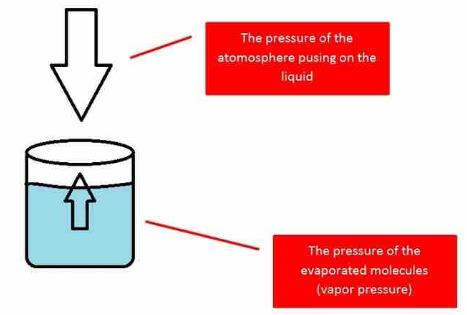Fig: When the pressure of the vapor equals the atmospheric pressure, the liquid will boil.

Since the addition of a non volatile solute causes a decrease in the vapour pressure of a solution, the boiling point of a solution is elevated as compared to the solvent i.e it must be heated to a higher temperature to make its vapour pressure equal to atmospheric pressure. Thus the solution boils at a higher temperature than the pure solvent.
If Tb˚ is the boiling point of the solvent and Tb is the boiling point of the  solution.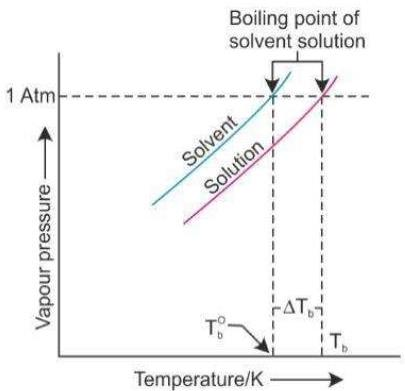Fig: Elevation in boiling point.

We can see from the figure that the solution needs to be heated to a higher temperature to make it boil.
The difference between the boiling points of the solution and the solvent is known as the elevation in boiling point (ΔTb).
Elevation of boiling point, (ΔTb)=
Boiling point of the solution - Boiling point of pure solvent = Tb -Tb˚
Experimentally, it is observed that elevation in boiling point is directly proportional to molal concentration of solute dissolved in definite amount of the solvent.
ΔTb ∝ molality
ΔT= K×  molality .....(1)
ΔTb = Kb × m
Where Kb is proportionality constant and is known as the molal boiling point elevation constant or the ebullioscopic constant. It has the units of degree kg mol-1 or K kg mol-1.
Molality can be written as
Molality (m) = Number of moles of solute/Mass of solvent in kg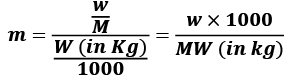w = mass of solute in grams
M = molecular wt of solute
W = mass of solvent in gram.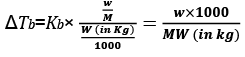Significance of Kb
Now if, m = 1 then,
ΔTb = Kb. Thus,
the molal elevation constant Kb is defined as the elevation of the boiling point when the molality of the solution is one i.e. when 1 mole of the solute is dissolved in 1 kg of the solvent, the elevation of boiling point is equal of Kb.

Example 1. A 5 percent aqueous solution by mass of a non-volatile solute boils at 100.15°C. Calculate the molar mass of the solute. Kb = 0.52 K kg mol -1
Solution.
The normal boiling point of water is 100°C.
The elevation in boiling point is 0.15°C.
A 5 percent solution means that in a 100 g solution 5 g of the solute is present.
This implies that the solvent is 95 g.
If the molar mass of the solute is M, then the molality of the solute in the  solution is,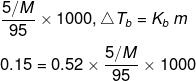M = 182.4 g/mol

Example 2. A solution of 12.5 g urea in 170 g of water gave a boiling point elevation of 0.63 K. Calculate the molar mass of urea, taking Kb = 0.52 K/m.
Solution.
Given: Mass of urea, w2 = 12.5 g
Mass of water, w1 = 170 g = 0.17 kg
Evaluation of boiling point, ΔTb = 0.63 K
Molar mass of urea, M = ?
Kb = 0.52 K m-1
Molar mass of the solute (urea) is given by: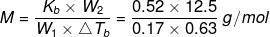= 60.7 g mol-1

Example 3. Calculate the molecular mass of a substance 1.0 g of which on being dissolved in 100 g of solvent gave an elevation of 0.307 K in the boiling point. (Molal elevation constant (Kb) = 1.84 K/m).
Solution.
We know that, ΔTb = , where n2 is the no. of moles of solute; w1 is the mass of the solvent in kg.
n2 = Mass of solute/Molar mass of the solute = 1.0 g/M and w1 = 100 g = 0.1 kg
Substituting these values, we get,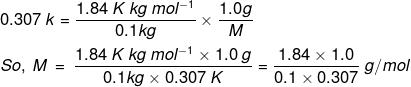= 59.9 g/mol

TRY YOURSELF!
Q.1. Pure benzene boils at 80°C. The boiling point of a solution containing 1 g of substance dissolved in 83.4 g of benzene is 80.175°C. If latent heat of vaporization of benzene is 90 cal per g, calculate the molecular weight of solute.
Ans. M = 189.72

Q.2. An aqueous solution of glucose boils at 100.01°C. The molal elevation constant for water is 0.5 K mol-1 kg. What is the number of glucose molecules in the solution containing 100 g water?
Ans. 1.2 × 1021 molecules

Offer running on EduRev: Apply code STAYHOME200 to get INR 200 off on our premium plan EduRev Infinity!

## Chemistry Class 12

121 videos|250 docs|199 tests

,

,

,

,

,

,

,

,

,

,

,

,

,

,

,

,

,

,

,

,

,

;